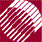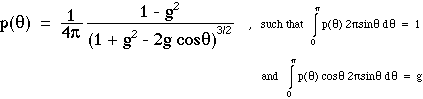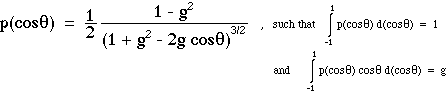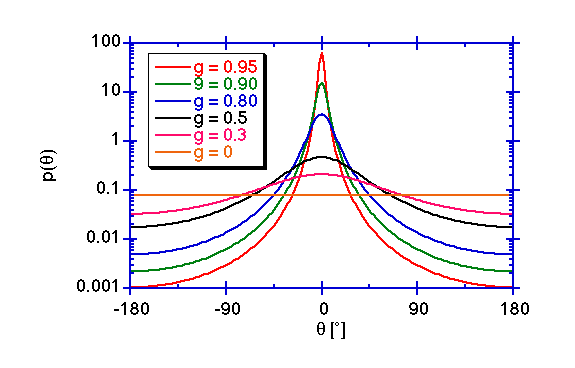ECE532 Biomedical Optics © 1998 Steven L. Jacques, Scott A. Prahl Oregon Graduate Institute

# Henyey-Greenstein scattering function

Henyey and Greenstein (1941) devised an expression which mimics the angular dependence of light scattering by small particles, which they used to describe scattering of light by interstellar dust clouds. The Henyey-Greenstein scattering function has proven to be useful in approximating the angular scattering dependence of single scattering events in biological tissues.

The Henyey-Greenstein function allows the anisotropy factor g to specify p(θ) such that calculation of the expectation value for cos(θ) returns exactly the same value g. In other words, Henyey and Greenstein devised a useful identity function. The Henyey-Greenstein function is:It is common practice to express the Henyey-Greenstein function as the function p(cosθ);A series of Henyey-Greenstein functions are shown in the following figure. The forward direction along the original photon trajectory is 0°. Scattering in the backward direction is 180°. The curve for g = 0 has a constant value of 1/4π.